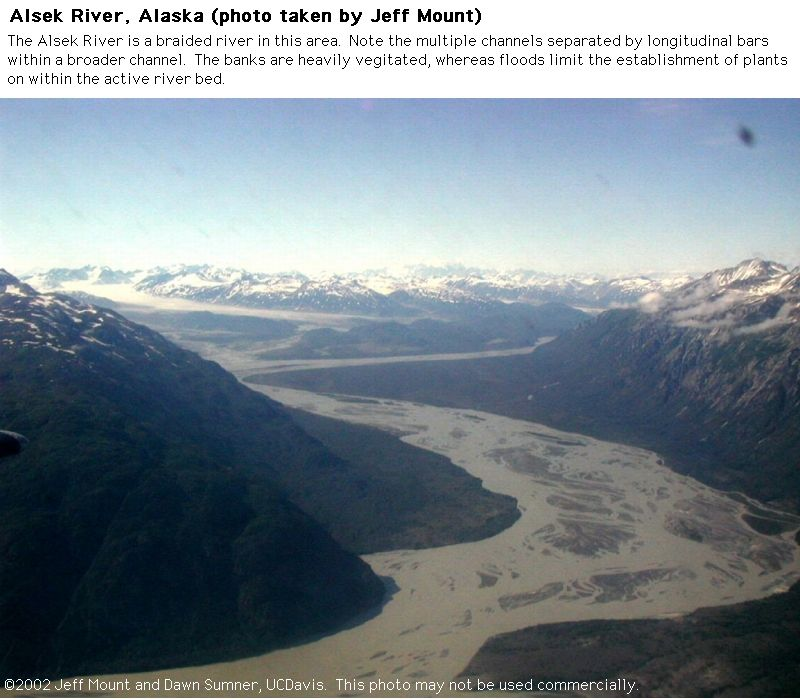# 6.1.5: Fluvial Environments (Chapter 9)Figure $$\PageIndex{1}$$: Fluvial Fan (Alaska)Figure $$\PageIndex{2}$$: Braided River (Alaska)Figure $$\PageIndex{3}$$: Braided River (Alaska)Figure $$\PageIndex{4}$$: Dunes migrating in a creekFigure $$\PageIndex{5}$$: Dunes migrating in a creekFigure $$\PageIndex{6}$$: Antidunes in a creekFigure $$\PageIndex{7}$$: Pebble scoursFigure $$\PageIndex{8}$$: Recent Alluvial Deposits (Terrace at Redrock State Park, CA)Figure $$\PageIndex{9}$$: Recent Alluvial Deposits (Terrace at Redrock State Park, CA)Figure $$\PageIndex{10}$$: Recent Alluvial Deposits (Death Valley, CA)Figure $$\PageIndex{11}$$: Channel Erosion Due to Changing Base Level/Flow Speeds (Death Valley, CA)Figure $$\PageIndex{12}$$: Ancient Trough Cross Stratification (two faces of the same rock)Figure $$\PageIndex{13}$$: Plan or Map View of Ancient Trough Cross StratificationFigure $$\PageIndex{14}$$: Ancient Trough Cross StratificationFigure $$\PageIndex{15}$$: Ancient Trough Cross StratificationFigure $$\PageIndex{16}$$: Fining Upward Fluvial CyclesFigure $$\PageIndex{17}$$: Small Fining Upward Fluvial CycleFigure $$\PageIndex{18}$$: Single set of cross strata in a planar bed​​​​​​​

Figure $$\PageIndex{19}$$: Recent Fluvial Deposits (Alsek River, Alaska)